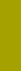﻿ DistributionsSand Texture Sedimentology

DISTRIBUTIONS

Frequency (probability) distributions

Both the mathematical statistics and the theory of probability specify a distribution as a mathematical function describing the amount (frequency, probability) of occurrences (dependent variable, graphically Y axis)  of an independent variable (graphically X axis). The independent variable may be a size of things — people, stars, particles/grains — or any related dispersity unit

Distribution moments

Geologists and (Earth scientists) have devoted extensive attention to the granularity of rocks, especially sedimentary ones. Sedimentologists have attempted to receive the most demanding details about the origin of the analyzed sediments and sedimentary rocks. However, they applied standard solutions of mathematical statistics without checking suitability for their own purposes. They started studying the first four moments of grain size distributions on hundreds of thousands of analyses.

First two — not four — distribution moments have physical meaning

However, only the first two moments have physical meaning, whereas the third moment, asymmetry, and the fourth one, kurtosis, are not magic numbers but only indicators of a non-normality (without expected physical background). This is why the studies using the first four moments and their percentile estimates in the second half of the previous century discredited the granularity of sediments and sedimentary rocks.

Normal (Gaussian) distribution on logarithms of the dispersity unit

The normal (Gaussian) distribution occurs only on logarithms of the dispersity unit, such as PHI particle size and PSI particle sedimentation velocity. However, the measured distributions show differences from a normal distribution. The overwhelming part of the differences is due to normal distribution mixing, either of the origin processes, of the source materials or both. The mixture normal components better characterize the origin than the bulk distribution characteristics, particularly when the component parameters are displayed in space (3D).

Distributions perfectly defined by distribution mixture components

Therefore, the best characteristics of a measured distribution is the determination of its mixture components: their parameters can perfectly define the measured distribution. However, not all components should be included. Within the particle size range of sand (0.05 - 4 mm), the maximum number of components is five.

Our program Shape™ includes a distribution decomposition up to five components with the Chi-Square (χ2) goodness of fit test.